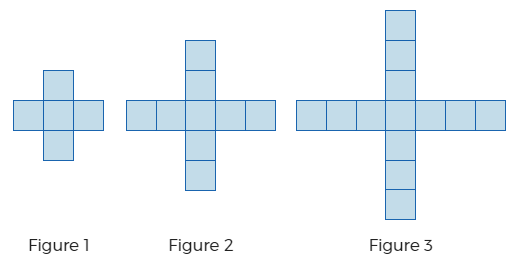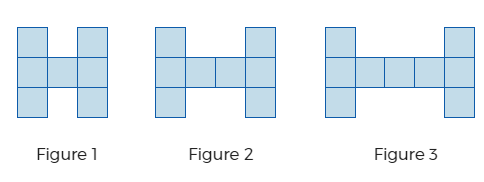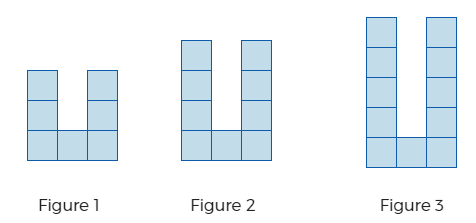# Exponents and Exponential Functions

## Objective

Write explicit rules for arithmetic sequences and translate between explicit and recursive formulas.

## Common Core Standards

### Core Standards

?

• F.BF.A.2 — Write arithmetic and geometric sequences both recursively and with an explicit formula, use them to model situations, and translate between the two forms. Modeling is best interpreted not as a collection of isolated topics but in relation to other standards. Making mathematical models is a Standard for Mathematical Practice, and specific modeling standards appear throughout the high school standards indicated by a star symbol (★). The star symbol sometimes appears on the heading for a group of standards; in that case, it should be understood to apply to all standards in that group.

• F.IF.A.3 — Recognize that sequences are functions, sometimes defined recursively, whose domain is a subset of the integers. For example, the Fibonacci sequence is defined recursively by f(0) = f(1) = 1, f(n+1) = f(n) + f(n-1) for n ≥ 1.

• F.LE.A.2 — Construct linear and exponential functions, including arithmetic and geometric sequences, given a graph, a description of a relationship, or two input-output pairs (include reading these from a table).

?

• 8.F.B.4

## Criteria for Success

?

1. Understand the difference between an explicit formula and recursive formula; understand that an explicit formula can generate a value of any term, without needing to know an existing term in the sequence.
2. Write explicit rules for arithmetic sequences and use them to find specific terms in the sequence.
3. Write recursive formulas for arithmetic sequences given an explicit formula, and vice versa.

## Anchor Problems

?

### Problem 1

The formula for an explicit rule for an arithmetic sequence is given by ${a_n=a_1+d(n-1)}$, where $d$ is the common difference and $n$ is the term number.

Write an explicit rule for the number of tiles in each pattern shown below.

a.b.c.### Problem 2

An arithmetic sequence is shown below.

${29,\space 22,\space15,\space8,\space1,\space…}$

1. Write a recursive formula to represent the sequence.
2. Write an explicit formula to represent the sequence.
3. Find the 10th term in the sequence.
4. Find the 100th term in the sequence.

### Problem 3

An arithmetic sequence is described by either a recursive or explicit rule below. If given a recursive rule, provide the explicit rule. If given the explicit rule, give the recursive rule.

a.   ${f(n)=6(n-1)+1}$, for ${n\geq1}$

b.   ${a_{n+1}=a_n-{3\over2}}$${a_1=10}$

## Problem Set

?

The following resources include problems and activities aligned to the objective of the lesson that can be used to create your own problem set.

Sequence: ${2,11,20,29,38,…}$
Recursive formula: ${a_{n+1}=a_n+9}$, where ${a_n=2}$